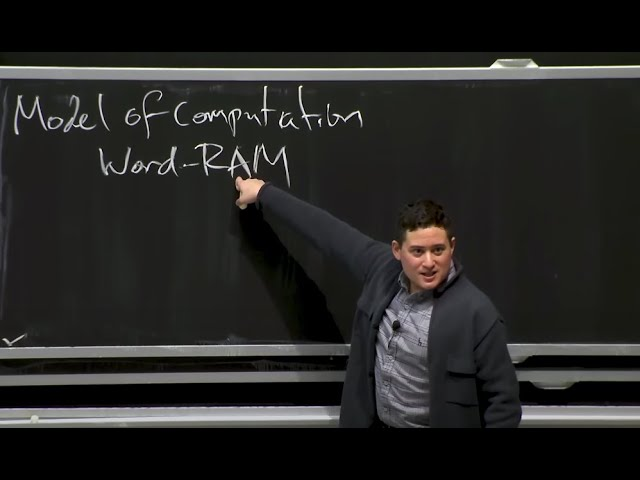# Master Computational Problem-Solving with Algorithms CourseTLDR The Introduction to Algorithms course teaches students how to solve computational problems, prove correctness and efficiency, and communicate ideas effectively through writing, using algorithms as recipes that take inputs and produce outputs, with their correctness proven through induction and recursion, and their efficiency measured by the number of operations needed to solve a problem, compared using asymptotic notation, and analyzed in terms of computational complexity and data structures.

## Key insights

• 💻
This course on Introduction to Algorithms aims to teach students how to solve computational problems.
• 📝
In theory classes, the focus is on being able to prove that the solutions are correct and better than other solutions, and being able to communicate those ideas to others, not just to a computer.
• 💻
An algorithm is a fixed size procedure that generates an output based on an input, and that output must be correct based on the problem specification.
• 💻
The bar for algorithm communication is set at the level where someone else can code it up and tell a computer how to do that thing.
• ⏱️
Efficiency of an algorithm is not just about how fast it runs, but also how fast it compares to other possible ways of approaching the problem.
• 📈
Logarithmic functions can be almost as good as constant functions in terms of efficiency for large input sizes.
• 🤖
The use of a word RAM as a model of computation in computer science allows for theoretical brevity and assumes constant time access to memory.
• 💾
The limitation on memory addresses with 32 bits is about 4 gigabytes, while with 64 bits it's something like 20 exabytes, which is more than enough for all data that Google stores on their servers.

## Q&A

• What is the Introduction to Algorithms course about?

The Introduction to Algorithms course teaches students how to solve computational problems, prove correctness and efficiency, and communicate ideas effectively through writing.

• How are algorithms used in solving computational problems?

Algorithms are used as recipes that take inputs and produce outputs, with their correctness proven through induction and recursion, and their efficiency measured by the number of operations needed to solve a problem.

• How can the correctness of an algorithm be proven?

The correctness of an algorithm can be proven through induction and recursion, with a base case needed for an inductive proof.

• How is the efficiency of an algorithm measured?

The efficiency of an algorithm is measured by counting the number of fundamental operations it needs to perform to solve a problem, compared using asymptotic notation.

• What topics are covered in the Introduction to Algorithms course?

The course covers data structures, sorting, shortest paths and graphs, and dynamic programming in order to solve algorithm problems.

## Timestamped Summary

• 📚
00:00
Introduction to Algorithms course teaches students how to solve computational problems, prove correctness, argue efficiency, and communicate ideas effectively through writing.
• 🧮
07:46
The proposed algorithm for the birthday problem is to maintain a record, interview students in order, check if their birthday is in the record, and return a pair if there is a match.
• 📝
13:13
An algorithm is like a recipe that takes an input and produces an output, and to prove its correctness for large inputs, we use induction and recursion.
• 💡
21:07
The algorithm for finding a match in a group of students involves checking all possibilities for the K prime plus 1th student by brute force.
• 💻
26:05
An algorithm's efficiency is measured by the number of operations it needs to solve a problem, and its performance is compared using asymptotic notation like big O, omega, and theta, with a linear time algorithm having a performance directly proportional to the size of the input.
• 💻
32:16
Different algorithms have varying running times, with polynomial being considered efficient, and computational complexity is measured using the RAM model with constant time access to memory.
• 💻
39:54
32-bit CPUs can only address 4GB of memory, while 64-bit CPUs can address up to 20 exabytes, and CPUs can only operate on a constant amount of information at once, important to consider when analyzing algorithms, with the first half of the class focusing on data structures that store large amounts of data and support different operations to make them faster.
• 👨‍💻
44:34
Learn to solve algorithm problems by reducing to known solutions or designing recursive algorithms, covering data structures, sorting, shortest paths, graphs, and dynamic programming.Report the articleThank you for the feedback